Courses

# Noise in Digital Communication GATE Notes | EduRev

## GATE : Noise in Digital Communication GATE Notes | EduRev

The document Noise in Digital Communication GATE Notes | EduRev is a part of the GATE Course Communication System.
All you need of GATE at this link: GATE

Noise in Digital Communication

In digital communication for better SNR, a matched filter is used whose impulse response h(t) is.

h(t) = S* (Tb – t)

where, * is represent complex conjugate

Tb = Bit duration

S(t) = Input signal to filter

Probability of error Pe is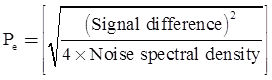where,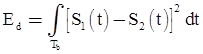Note: N/2 is two sided noise power spectral density.

Probability of Error The Probability of error for different digital modulation schemes is given below

Probability of Error Different Types of Digital Modulation Schemes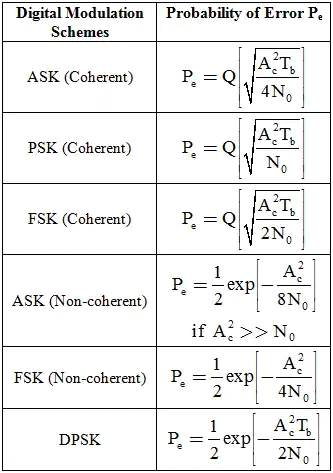• In case of FSK f1 and f2 are choose such that f1 = mfs and f­2 = kfs′ where m and are integers.
• Bandwidth efficiency for PSK is: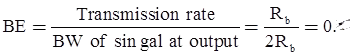Noise:

In electrical-terms, noise may be defined as an unwanted form of energy which tend to interfere with the proper reception and reproduction of transmitted signals. Conveniently noise can be classified as:

• External noise
• Internal noise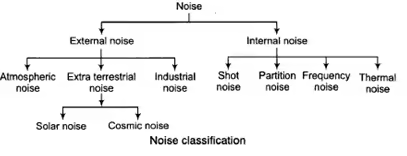Noise Analysis in Communication System:

The noise analysis can be done in communication system by calculating the following terms

Figure of Merit:

Noise analysis in Continuous Wave (CW) modulation is carried out in the form of a parameter known as figure of merit denoted by γ. This parameter figure of merit γ is the ratio of output signal-to-noise ratio to the input signal-to-noise ratio of the receiver.

Signal to Noise Ratio (SNR):

It is defined as ratio of signal power to noise power.

In-phase noise component: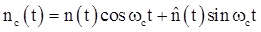Where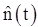is the Hilbert transform of n(t)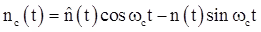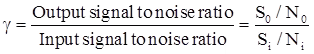where, n (t) represents the filtered noise

Total noise power (N) = White noise power spectrum density x Bandwidth

or

N= (n/2) * Bandwidth

Thus, the noise has a gaussian distribution.

• The effect of channel noise may be obtained by simple addition of signal x(t) and noise n (t).
• The noise performance depends on the relative magnitudes of the signal and noise.

Effect of Noise on a Baseband System

SNR is given by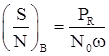Where, PR = is received signal phase, N0 = two sided noise spectral density, and ω = Message signal bandwidth.

SNR of baseband system: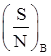Effect of Noise on DSBSC AM

For coherent receiver, SNR at the output is: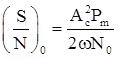where, Pm = Message signal power, Pc = Carrier signal amplitude, and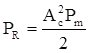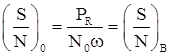In DSBSC, the output SNR is the same as the SNR for a baseband system. Therefore DSBSC does not provide any SNR improvement over a baseband communication system.

Effect of Noise on SSB AM

For coherent receiver, SNR at the output is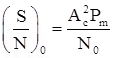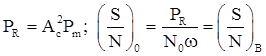SNR in case of SSB is same as that of DSBSC and baseband system.

Effect of Noise on Conventional AM

For coherent receiver, SNR at the output is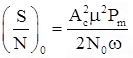where, Ac = Amplitude of carrier wave, μ = Modulation index, and Pmn = Normalized message signal power.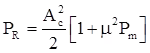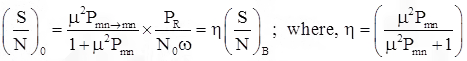SNR of conventional AM is always less than the SNR of a baseband system.

Effect of Noise on Angle Modulation

Noise spectral density at the output of angle modulation receiver is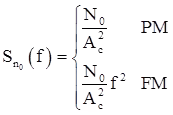where, N0/2 is two sided power spectral density of noise.

• Effect of noise is independent of frequency for PM systems.
• Effect of noise is more at higher frequencies and less at small frequencies for FM systems.

For angle modulation system, SNR at output is

for PM: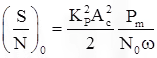where, Pm = message signal power

For FM :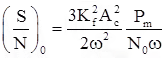where, (A2c /2) received signal power Pr.

For PM :where, β= modulation index of PM system.

For FM:where, β= modulation index of FM system.

With increase in β without increasing the transmitter power we can increase SNR at output. Increasing β will increasing the bandwidth requirement for transmission so we can increase SNR by increasing bandwidth.

Note: In both PM and FM systems, output SNR is proportional to the square of modulation index.

Offer running on EduRev: Apply code STAYHOME200 to get INR 200 off on our premium plan EduRev Infinity!

10 docs|12 tests

,

,

,

,

,

,

,

,

,

,

,

,

,

,

,

,

,

,

,

,

,

;